# IQ Test Labs

Brainteasers > Pictures > Triangles• Spot the triangles within the triangles!
• There are triangles of all sizes in the figures.

1. Count the triangles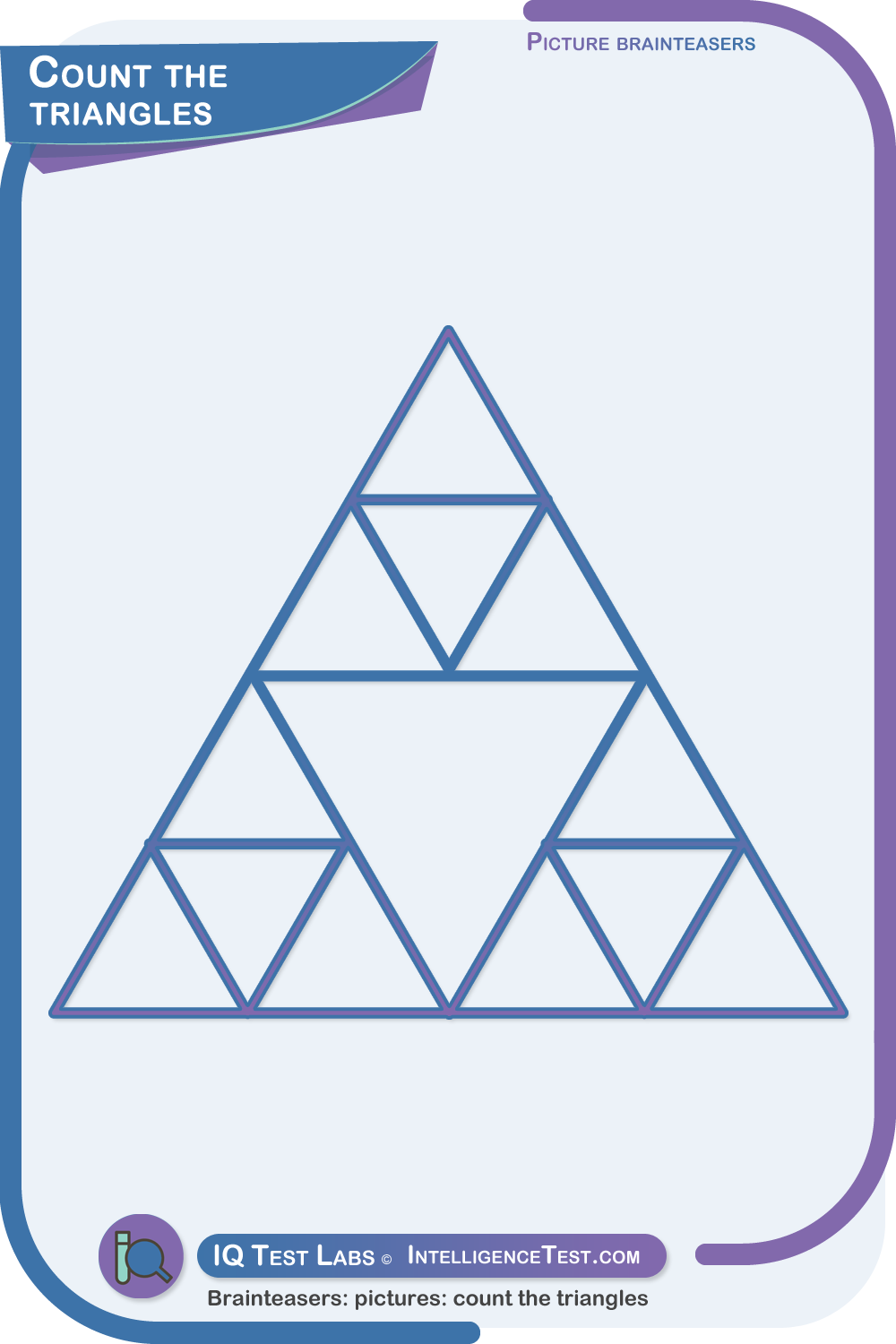There are 17 triangles.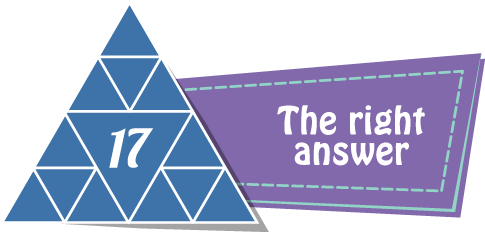2. Count the triangles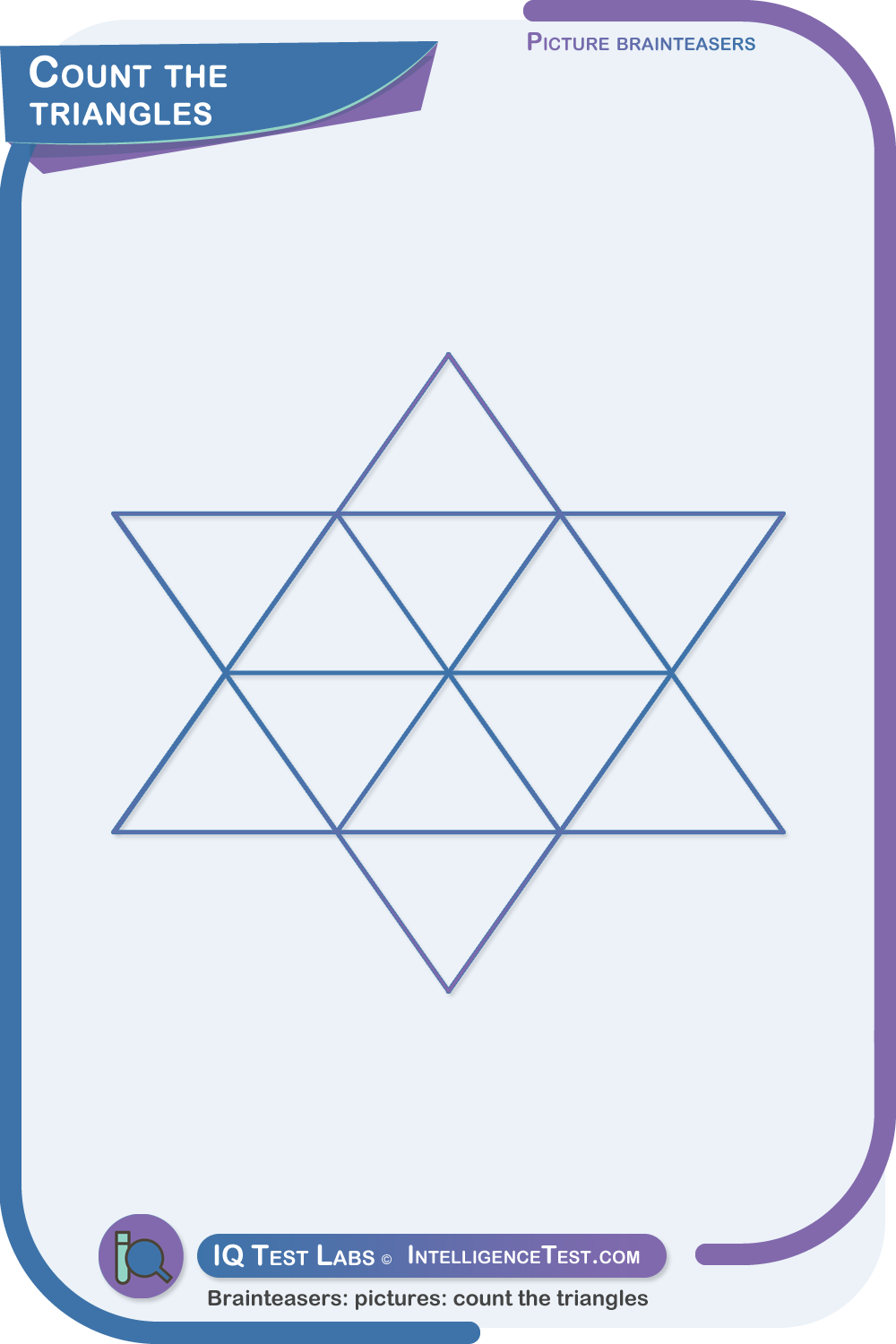There are 20 triangles.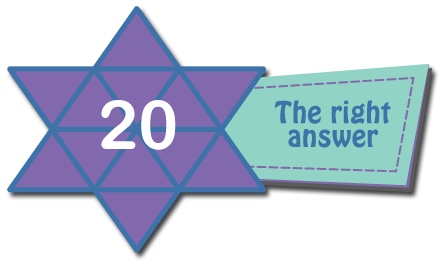3. Count the triangles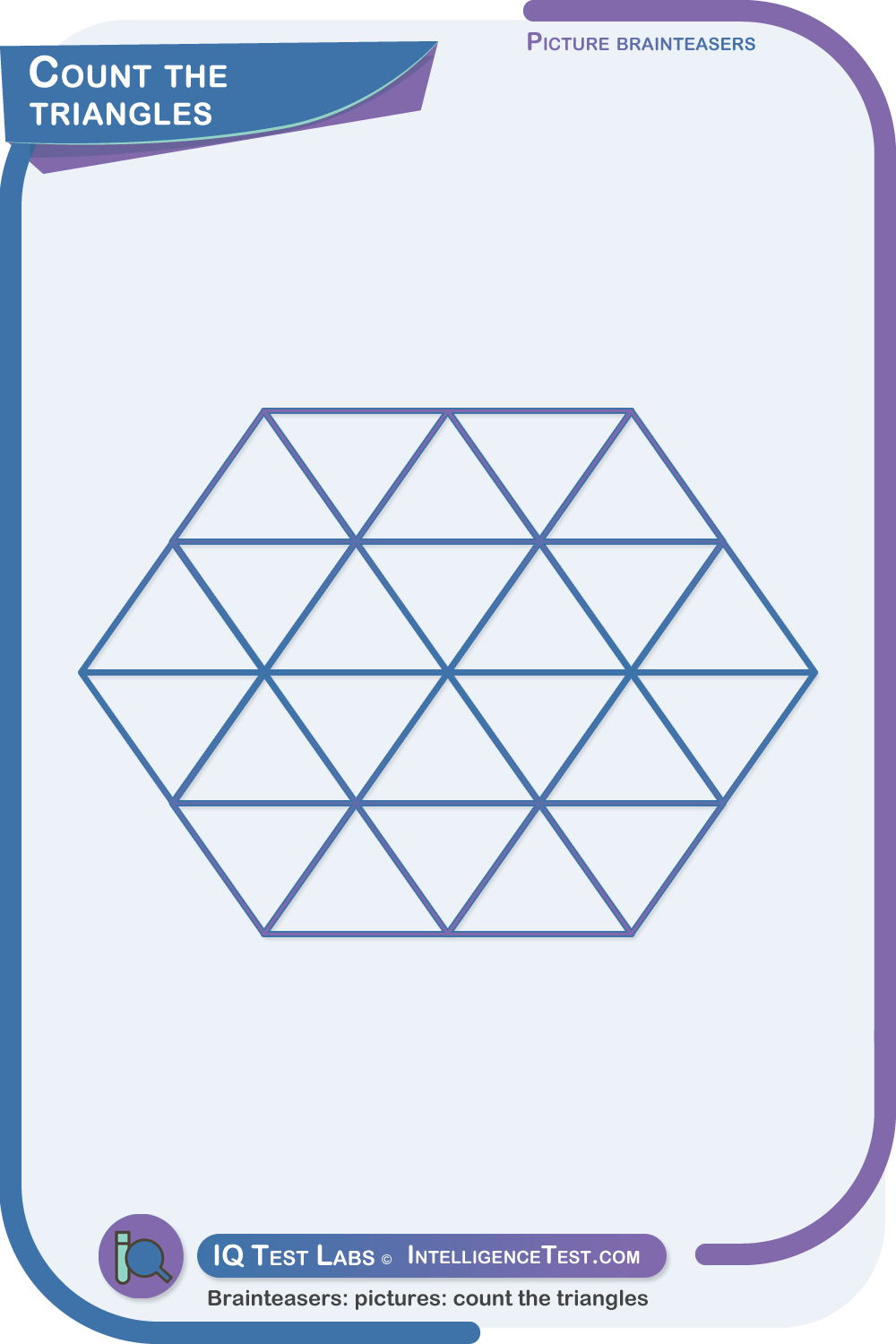There are 38 triangles.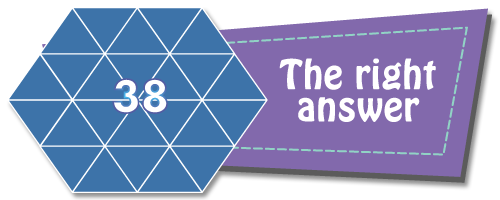4. Count the triangles.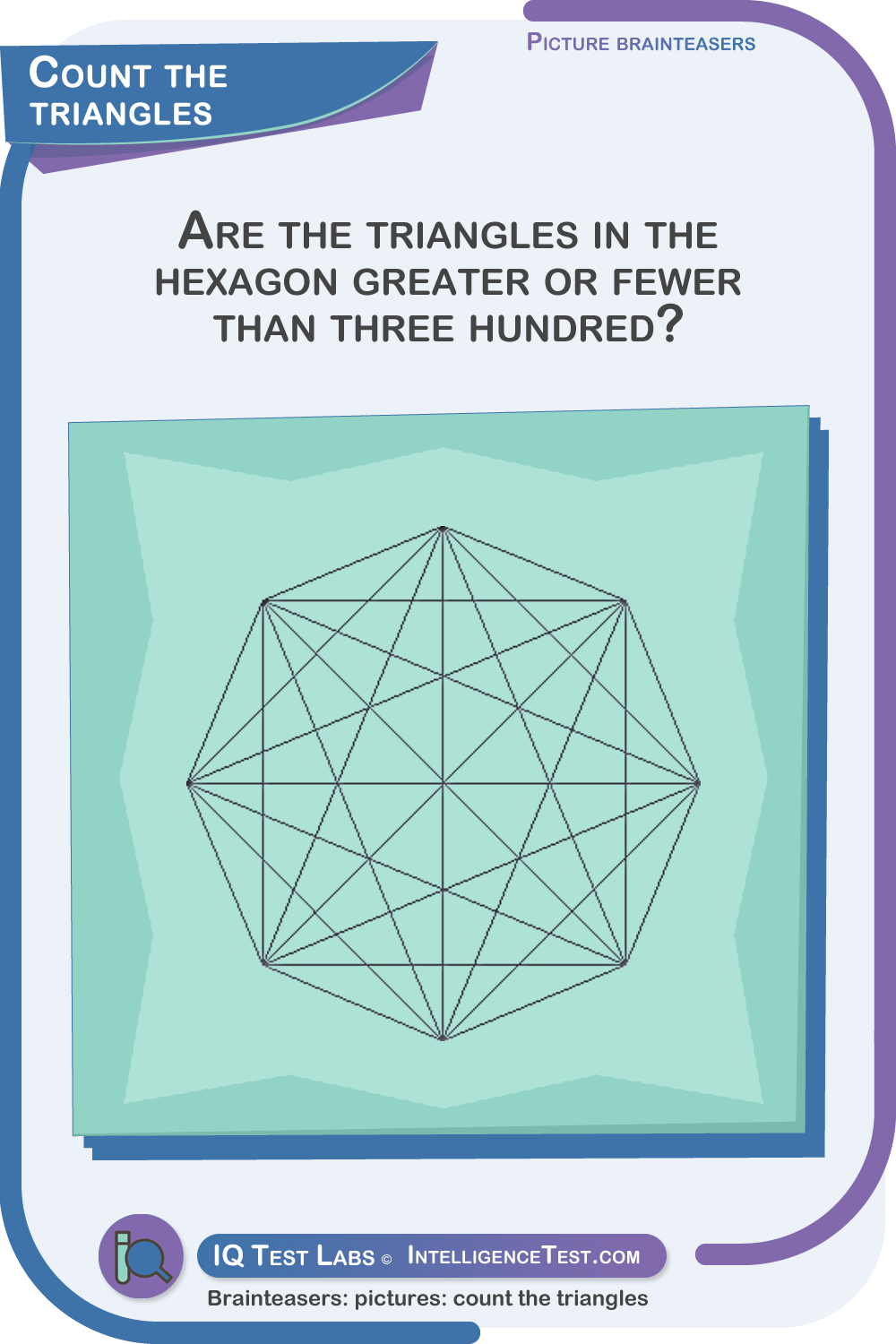There are 632 triangles.